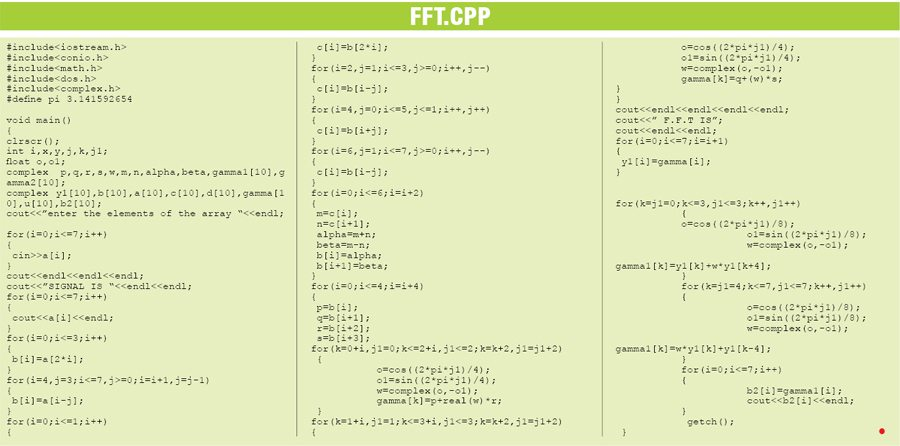Saturday, September 30, 2023

# Implementation of Fast Fourier Transform Using C++

Here is a program to compute fast Fourier transform (FFT) output using C++. -- Ahlad Kumar

Here is a program to compute fast Fourier transform (FFT) output using C++.

FFTs are of great importance to a wide variety of applications including digital signal processing (such as linear filtering, correlation analysis and spectrum analysis) and solving partial differential equations to algorithms for quick multiplication of large integers. Its efficient computation is a topic that has received considerable attention by many mathematicians, engineers and applied scientists.

In the field of signals and systems, there are two ways of looking at any complex waveform like square wave, saw-tooth or a practical voice signal: time domain and frequency domain. Depending upon the need to solve a problem or know the answer of some abrupt behaviour in a system response, we try to switch in between these two domains. Sometimes, a signal in time domain itself gives answers to many questions but sometimes we need frequency domain to get the answers. For example, we need frequency domain to know that a voice signal contains frequency content up to 20 kHz and not beyond that, and also to know that transmitting it over a telephone requires voice frequency up to 3.4 kHz and the rest of it is of no use.

Here we show an example to explain what it means to look at a signal in two domains. Fig. 1 shows a square signal in time domain and Fig. 2 shows its frequency domain.

We can see from these two figures that frequency domain gives more insight into a signal than time domain. The peaks in Fig. 2 show the frequency content present in a square wave. The highest peak corresponds to the fundamental frequency, while the other peaks are called harmonics. By seeing such a frequency-domain plot of voice signals researchers came out with a decision that up to 3.4 kHz of frequency content is useful, while the other harmonics have a very small amplitude that can be neglected. The discussion and arguments so far are equally valid for discrete signals as well.

Fast Fourier transform
Discrete Fourier transform (DFT) is the way of looking at discrete signals in frequency domain. FFT is an algorithm to compute DFT in a fast way. It is generally performed using decimation-in-time (DIT) approach. Here we give a brief introduction to DIT approach and implementation of the same in C++.

DIT algorithm. Computational efficiency in the evaluation of DFT is achieved by decomposing the sum of ‘N’ terms into sums containing fewer terms. These decomposition schemes give rise to highly efficient algorithms known as fast Fourier transforms. These techniques are based on the ways in which ‘N’ can be factored. For most part we consider values of ‘N’ that are integral powers of ‘2,’ that is:
N=2m

where ‘m’ is a positive integer.

Here we have taken m=3, so N=8. The signal flow graph in Fig. 3 shows a useful representation of a system of equations.

Here we present C++ code for implementing 8-bit FFT of a given input sequence using DIT algorithm discussed in Fig.3.

Software program
The software, written in C++, is compiled using Turbo C++ Version 3.0. It was tested on Windows XP SP3 machine. Run the FFT.exe file and enter each signal element of an array followed by pressing Return/Enter key. Up to eight input elements can be entered.Fig. 4 shows the screenshot of program output. Here the coefficient of input ‘x’ is (1, 3, 5, 7, 9, 11, 13, 15). The output is given in (A, B) format, which indicates ‘A’ is real and ‘B’ imaginary component of the complex number. In order to verify the correctness of our code, we calculated FFT of the same sequence (1, 3, 5, 7, 9, 11, 13, 15) in MATLAB as shown in Fig. 5.

It can be seen that MATLAB outputs the same FFT coefficients as our C++ code, which proves the correctness of our code.

The author is M. Tech from ABV-Indian Institute of Information Technology and Management, Gwalior, and B.Tech from Jamia Millia Islamia in New Delhi. Currently, he is doing Ph.D from University of Malaya, Malaysia. His research interests include low-voltage analogue design and mixed-signal design

1.Dralee

•Abhimanyu Rathore

Kindly check now.

2.Yash Patil

Source code link is broken, shows error 404.11

3.shabir

hi sir i want a code of this program and propsal please sir help me i request u okease its my lose and win problem please sir

•EFY Team

The source code is present on the 2nd page of the article.

4.bushra

Can anyone help me with 3d fft ?

•EFY Team

5.yash

can i say something, your code is full of errors man.

6.mona

the source code is still not available

•EFY Team

Hi, the source file is updated. You can download the Source Code from the article.

7.Mujahid Ali

Can someone pls explain the source code. it would be great favor from your side..Thanks